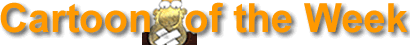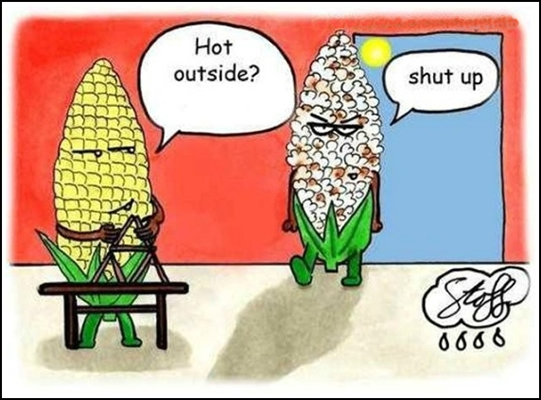Previous Cartoons of the Week

keyboard_arrow_left
keyboard_arrow_right

•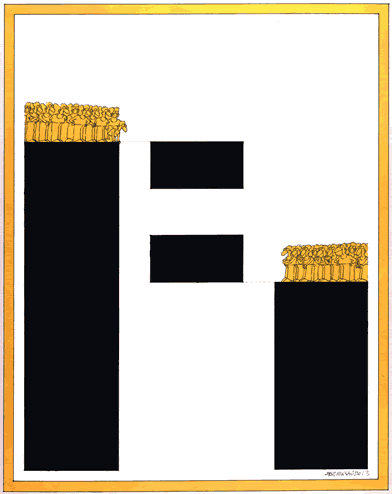{"ops":[{"insert":"\n"}]}

•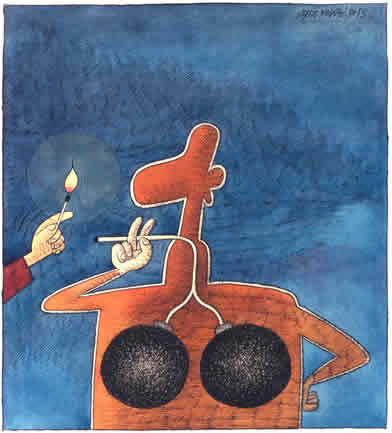{"ops":[{"insert":"\n"}]}

•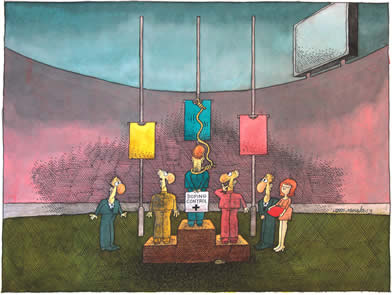•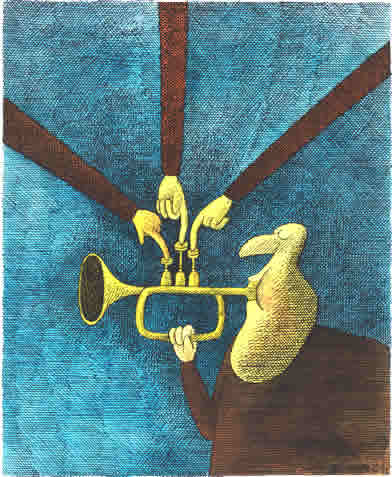{"ops":[{"insert":"\n"}]}

•{"ops":[{"insert":"\n"}]}

•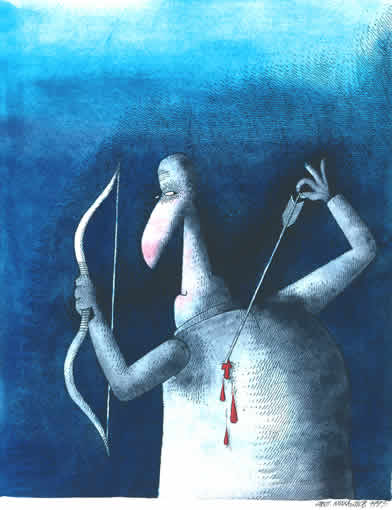{"ops":[{"insert":"\n"}]}

•{"ops":[{"insert":"\n"}]}

•{"ops":[{"insert":"\n"}]}

•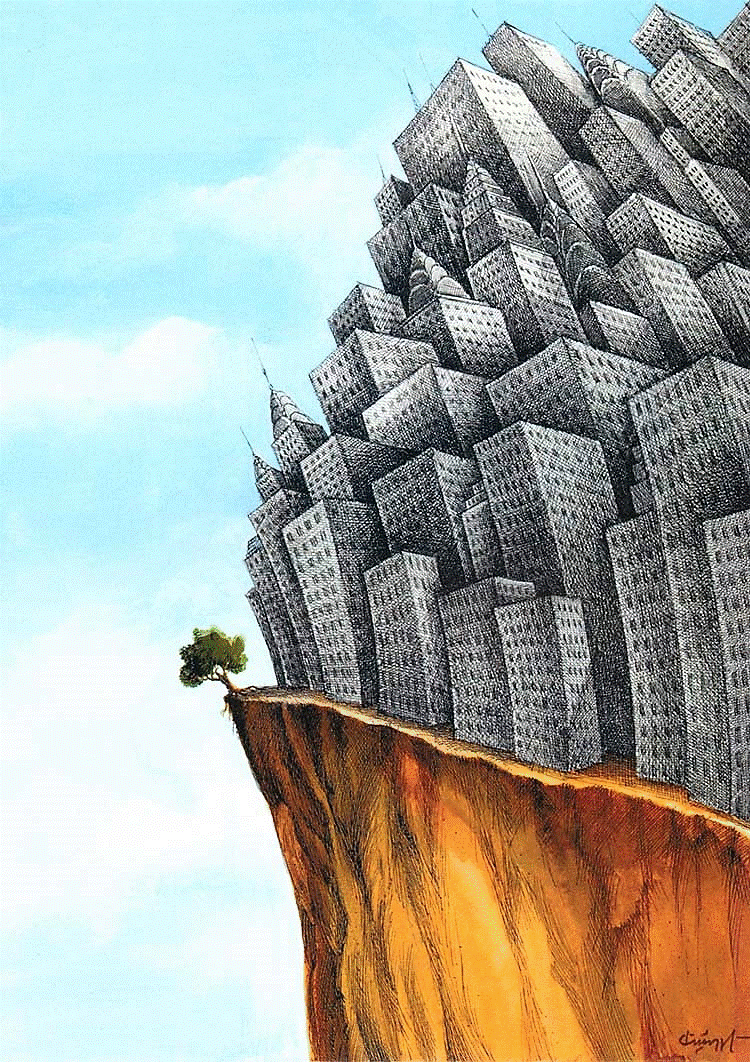{"ops":[{"insert":"\n"}]}

•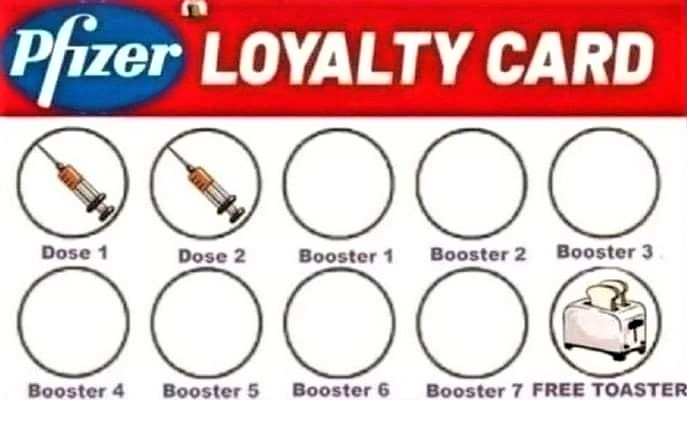{"ops":[{"insert":"\n"}]}

•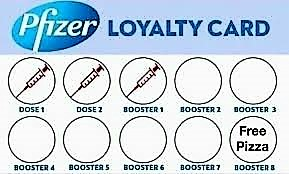{"ops":[{"insert":"\n"}]}

•{"ops":[{"insert":"\n"}]}

•{"ops":[{"insert":"\n"}]}